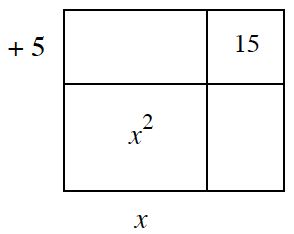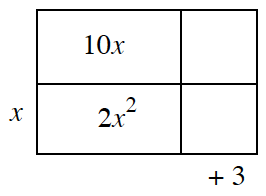Home > INT2 > Chapter 2 > Lesson 2.2.1 > Problem2-55

2-55.

Copy each of the area models below and fill in the missing dimensions and areas. Then write the area as a product equivalent to the area as a sum. Homework Help ✎

 a.Answer (a):$x^² + 8x + 15 = (x + 3)(x + 5)$ b.Answer (b):$2x^² + 13x + 15 = (2x + 3)(x + 5)$